# Solution assignment 05 Logarithmic functions and graphs

### Assignment 5

Sketch the graph of the following logarithmic function: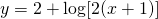### Solution

The logarithm: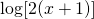is defined for: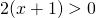and its graph intersects with the X-axis if: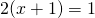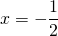We can verify the result in the figure.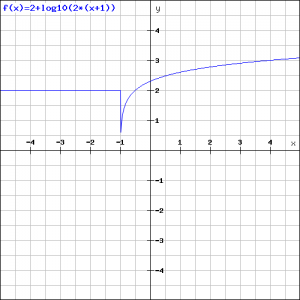The graph is only valid for.

0